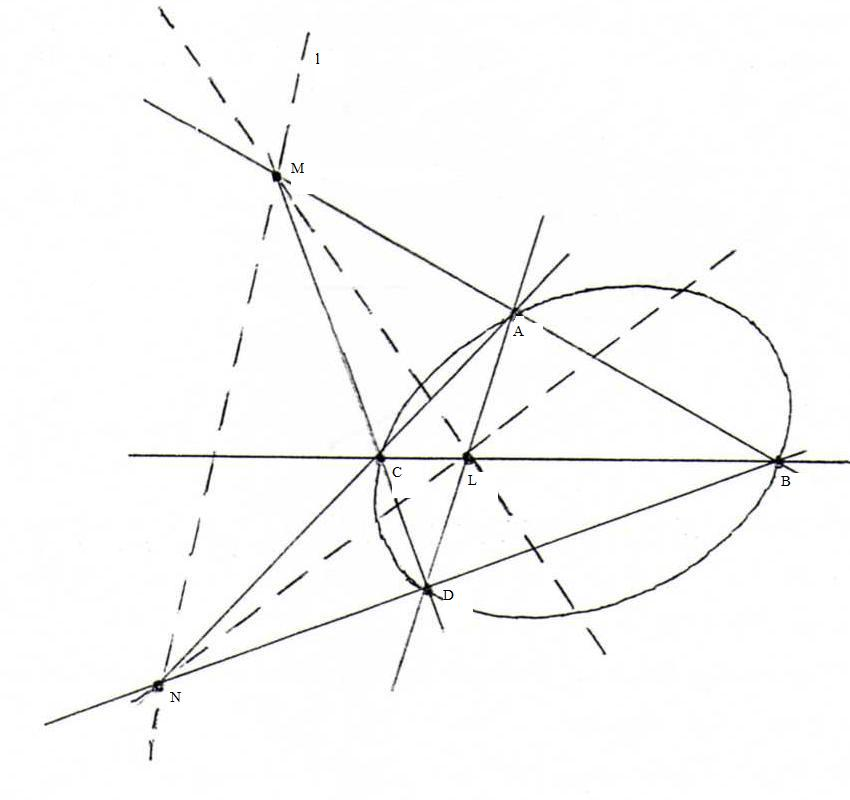### COURSE OF PROJECTIVE GEOMETRY

§ 22:

O83
Choose two points A and B on k, and let M be the intersection point of AB and l.Choose a new point N on l, and let C be the intersection point of AN and k, and let D be the intersection point of BN and k .
Then L is the diagonal point of the complete quadrangle ABCD that is not lying on l.